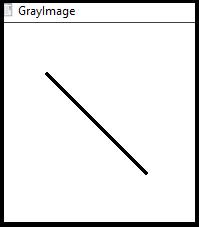# How to draw a line in OpenCV using C++?

To draw a line we need two points-the starting point and ending point. We also require a canvas to draw the line.

Using OpenCV, the matrix in our canvas, we need to define the line's starting and ending points. We require to assign a color to the line as well. The thickness of the line has to be explained too. If we want to draw a line using OpenCV, we need to declare a matrix, two points, and color and line thickness.

Using OpenCV we have to include <imgproc.hpp> header because line() function is defined in this header.

The basic syntax of this method is as follows −

## Syntax

line(whiteMatrix, starting, ending, line_Color, thickness);

The following program shows how to draws a line on an image in OpenCV −

## Example

#include<iostream>
#include<opencv2/highgui/highgui.hpp>
#include<opencv2/imgproc/imgproc.hpp>
using namespace cv;
using namespace std;
int main() {
Mat whiteMatrix(200, 200, CV_8UC3, Scalar(255, 255, 255));//Declaring a white matrix//
Point starting(50, 50);//Starting Point of the line
Point ending(150, 150);//Ending Point of the line
Scalar line_Color(0, 0, 0);//Color of the line
int thickness = 2;//thickens of the line
namedWindow("GrayImage");//Declaring a window to show the line
line(whiteMatrix, starting, ending, line_Color, thickness);//using line() function to draw the line//
imshow("GrayImage", whiteMatrix);//showing the line//
waitKey(0);//Waiting for KeyStroke
return 0;
}

## Output